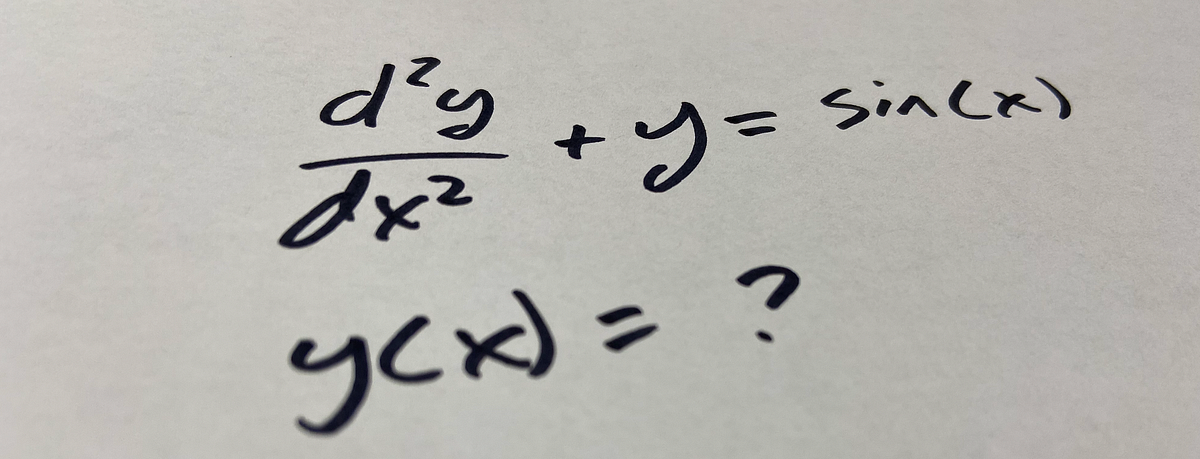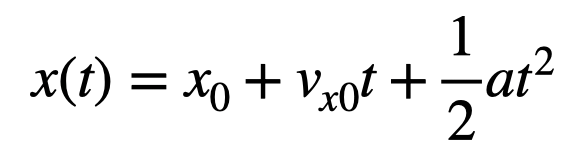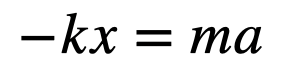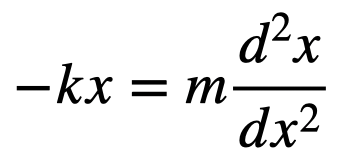# How Do You Solve a Differential Equation With Python?I guess I should start with a very basic explanation of differential equations. Of course you probably know something about equations, right? This is the equation for the motion of an object with a constant acceleration. Notice that my examples are oriented towards physics — because that’s what I like.

I guess I should start with a very basic explanation of differential equations. Of course you probably know something about equations, right? This is the equation for the motion of an object with a constant acceleration. Notice that my examples are oriented towards physics — because that’s what I like.This shows a relationship between two variables — in this case, the position (x) and time (t). Note: the acceleration is just a constant in this case. Great.

Now suppose that I have a mass oscillating back and forth while connected to a spring (horizontally with no friction). In that case, I have the following relationship between position (x) and the acceleration (a).Here, k is the spring constant and m is the value of the mass. But in this case, you can see that the acceleration is NOT constant. It changes as x changes. Also, the acceleration is the derivative of the velocity (with respect to time) and the velocity is the derivative of the position. So, maybe I should write the equation as:Now we have a relationship between a variable (x) and a derivative (technically a second derivative). This is a differential equation. There are many methods to solve differential equations — such as separation of variables, variation of parameters, or my favorite: guessing a solution.

But I’m not going to do any of those. Instead, I am going to solve a differential equation numerically.

## Guide to Python Programming Language

Guide to Python Programming Language

## Python Programming: A Beginner’s Guide

Python is an interpreted, high-level, powerful general-purpose programming language. You may ask, Python’s a snake right? and Why is this programming language named after it?

## Python Hello World Program - Create & Run Your First Python Program in PyCharm

Python Hello World Program - Your first step towards Python world. Learn how to create the Hello World Python program in PyCharm.

## Python Programming Tutorials For Beginners

Python Programming Tutorials For Beginners

## How to Remove all Duplicate Files on your Drive via Python

Today you're going to learn how to use Python programming in a way that can ultimately save a lot of space on your drive by removing all the duplicates. We gonna use Python OS remove( ) method to remove the duplicates on our drive. Well, that's simple you just call remove ( ) with a parameter of the name of the file you wanna remove done.